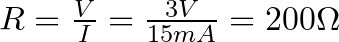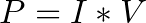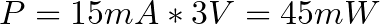# Current Limiting Resistor

A current limiting resistor is a resistor that is used to reduce the current in a circuit.

A simple example is a resistor in series with an LED.

You would usually want to have a current limiting resistor in series with your LED so that you can control the amount of current through the LED.

If too much current is going through your LED, it will burn out too fast. If too little current is going through it, it might not be enough to lit the LED.

## Calculating the necessary resistor value

Check the datasheet of your component to find voltage drop and the appropriate current for your LED.

If you can’t find a datasheet, you can test it.

Connect the LED and a resistor in series to a variable voltage source. Start at 0 volts and increase the voltage gradually until the LED lit up.

Measure the voltage over the LED and the current going through.

Let’s say the LED needs 15 mA and has a voltage drop of 2 volts. You have a 5 V power source that you would like to power it with. Which resistor value do you need?

To find the resistor value, we start with finding the voltage drop over the resistor. Since there is a 2 volt drop over the LED, there will be a 3V drop over the resistor.

Ok, so we have 3V and we want to have 15 mA going through the resistor and the LED.

To find the necessary resistor value we use Ohm’s law.this gives usSo the necessary value for the current limiting resistor is 200 Ohms.

## Choosing the right resistor

So you know that you need a 200 Ohm resistor.

But when you look for components you find that there are several different types of resistors.

Well, the only thing you need to be aware of is the wattage rating of the component. How much effect can the resistor handle?

So you need to figure out how much effect that will be dissipated in your resistor.

To find this you use the following formula for calculating powerIt states that power equals the current multiplied with the voltage. So we getThis means your resistor must be able to handle at least 45 mW.

Usually, most resistors handle from 250 mW and up, so it will be easy to find a suitable resistor.

Return from Current Limiting Resistor to Electronic Schematics

## More Resistors Tutorials

### 52 thoughts on “Current Limiting Resistor”

1. This is great! Gives the detail we need in easy speak! Thanks!

2. Thanks. Simple and great tutorial for a beginner.

3. what a nice post. i love it. thanks

• Thanks for the explanation. You kept the explanation nice and simple which is good for the beginners.

4. Wow simple with a great value…….hats off….

5. Very Nice! I’m really impressed with these topics!!

6. very nice answer sir awesome which is really needy for learners.
Sir, now in above you explained the conditions of requirement of resistor in circuit, i am very interested to know what are the conditions that makes to use capacitor & inductor in circuit & how to calculate the exact value of capacitor & inductor.

Regards,
Prashant.

7. Hi Sir,

Please explain me what is the meaning of Equivalent Series Resistance(ESR) & Its importance in the circuit.

Regards,
Prashant.

8. It’s not important

9. What will happen if you use a higher value of resistor ?? .. I used a 9v battery 390ohm resistor to light up an led and it still works for 1k and even 10k .. Pls explain

• If you use a higher value resistor, you get less current through your LED and your LED will be less bright.

Cheers!
Oyvind

• Sir
In a 5watts Led drive circuit there are 4 tiny flat black resistors marked as R1, R2,R3,and R4′.on top of each resistor,400odds values are marked.
I want to know i f that’s Ohm values or resistance power of each?
2question
Can I remove one of them from the circuit board so as to increase the brightness?
Thanks sir

10. It would be nice if Your teaching wouldn’t assume that we dommies new what these formulas were about , As You teach add notations to Your formulas !, to explain them , I no it takes up more space ! Pictures fill in the Q?????

11. I really love this

12. Thank you for this. Now I can order some resistors to test :)

13. thank you so much!!!!

14. What if a have 12v input and needs to light up a 10v led.what resistor will i need and the type of transisitor to be use?

Hoping you could help me.tnx..

15. Explaining scientifc knowledge (or fact) easy is not an easy thing to do. Great explanation and an example. What if I change + and – of the battery? Does an resistor still function what it has to do (does it reduce properly the voltage needed to the LED?)

• Resistor are not polarity sensitive but LEDs are it will burn out the LED.

16. Very easy to understand,thank you.

17. Nice sir give us information ike this????????????????☺????????☝????

18. Great explanation and very easy to understand as a beginner like me.

19. i salute you sir thanks this webpage i learn much more

20. Fantastic!Fantastic!Fantastic!

I loved the way of explanation…Great work…

So easy…

Thanks a lot …

21. very easy to understand even im not very understand english…tq pal…

22. Beautiful explanation understood in my life…. thanx bro

23. I liked the explanation as it was kept simple. Good for those beginners like myself

24. Im having a circuit for 12 volt and 240 mA.
Power source: 12 volt 5 amps transformer.

What value of resistance should i place?

Thanks.

25. This is exactly what I have been looking for since the age of 6(the first time I shocked myself, plugging in an Oreck vacuum for my grandma). It was then when my life’s mission to learn everything I can about this invisible monster…. Now I can plot my revenge…..⚡💣💥🤳🍻🍾

26. If we want the current through the entire circuit though shouldn’t we use 5V/15mA instead of 3V?

• 5V is the voltage drop across both the resistor and the LED. But only 3V out of the 5V from the battery drops across the resistor (if we are using a 2V LED). So we need to use 3V.

27. Thanks for the post. But i have a question that has been bothering me for long. Why don’t we put the power supply current into consideration?

• That’s because the current specified on the power supply is the maximum current, not the actual current. The actual current flowing is decided by the circuit, not the power supply.

28. Thanks for the lessons

29. More strength to your elbow

30. So a LED that has a Voltage drop V_drop = 1.8V / 20mA
is a load on the circuit and thus has a resistance: in this case 90ohm
this is independent of voltage as long as voltage is over 1.8V (right?)

Seeing that a resistor is also a load and limits (and conducts) a current; is it even needed if we get the voltage to the LED right at V-drop (perfect world)?

But for a given 5V voltage you have a spare 3.2V to get rid of. All voltage must be removed?
So this is your equation to figure out a resistor (in a perfect world): (5.0 – 1.8V) / 0.020A
or 160ohm.
Resistors just waste heat so we should minimize their ohms, right? So we should minimize
voltage every chance we have, right?

• Yes to all your questions =)

31. What if my power source voltage matches the specified LED voltage, let’s say they are both 3V. Do I still need a resistor?

• Then it’s okay to skip the resistor.

32. Wait, so how did you get to 3V across the resistor? You only measured the current and voltage for the LED. Is there a connection between the 2V in the LED and 3V in the resistor? I don’t get it.

• There is 5V in total. Since 2V drops across the LED, the remaining 3V must drop across the resistor, since there are no more components in series.

33. Hi. Love the site. I suggest being more fastidious with your units. Make a note when using milliamps or milliwatts, because the formulas are in amps and watts. Conversions are easy, as you know, but people can get tripped up with that.

• Thanks, I’ll try to remember that!

34. Great, thank you , cool

35. If we used 100 ohm resistor instead of 160 ohm , will current increase in this circuit or with same current only voltage increase across led?
If current is same as 20 ma 2 v will be dropped across resistor and led will get 3 v or
If voltage drop same 3v/100 ohms .03 amp or 30 ma will flow through the circuit
Actually what will happen please explain….